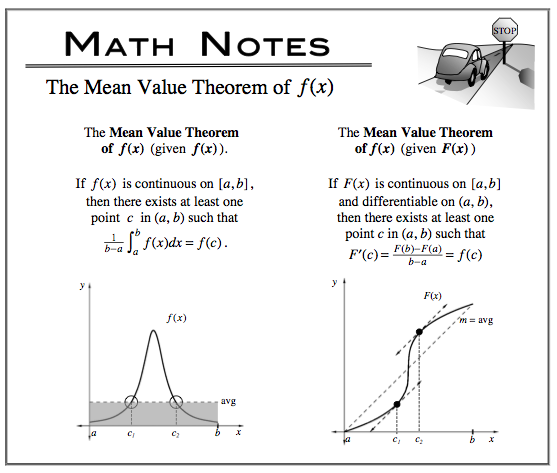### Home > CALC > Chapter 7 > Lesson 7.1.2 > Problem7-19

7-19.

It so happens that Hustling Harry's grade in the class at any week $t$ during the semester is calculated by $g(t) = 46\operatorname{cos}\frac{t}{10} + 10\operatorname{sin}\frac{t}{2} + 40$. At what point during the $18$-week semester was Harry's grade equal to his average grade for the semester?

This is an application of the Mean Value Theorem.

Show setup and steps. $t ≈7.630$ weeks